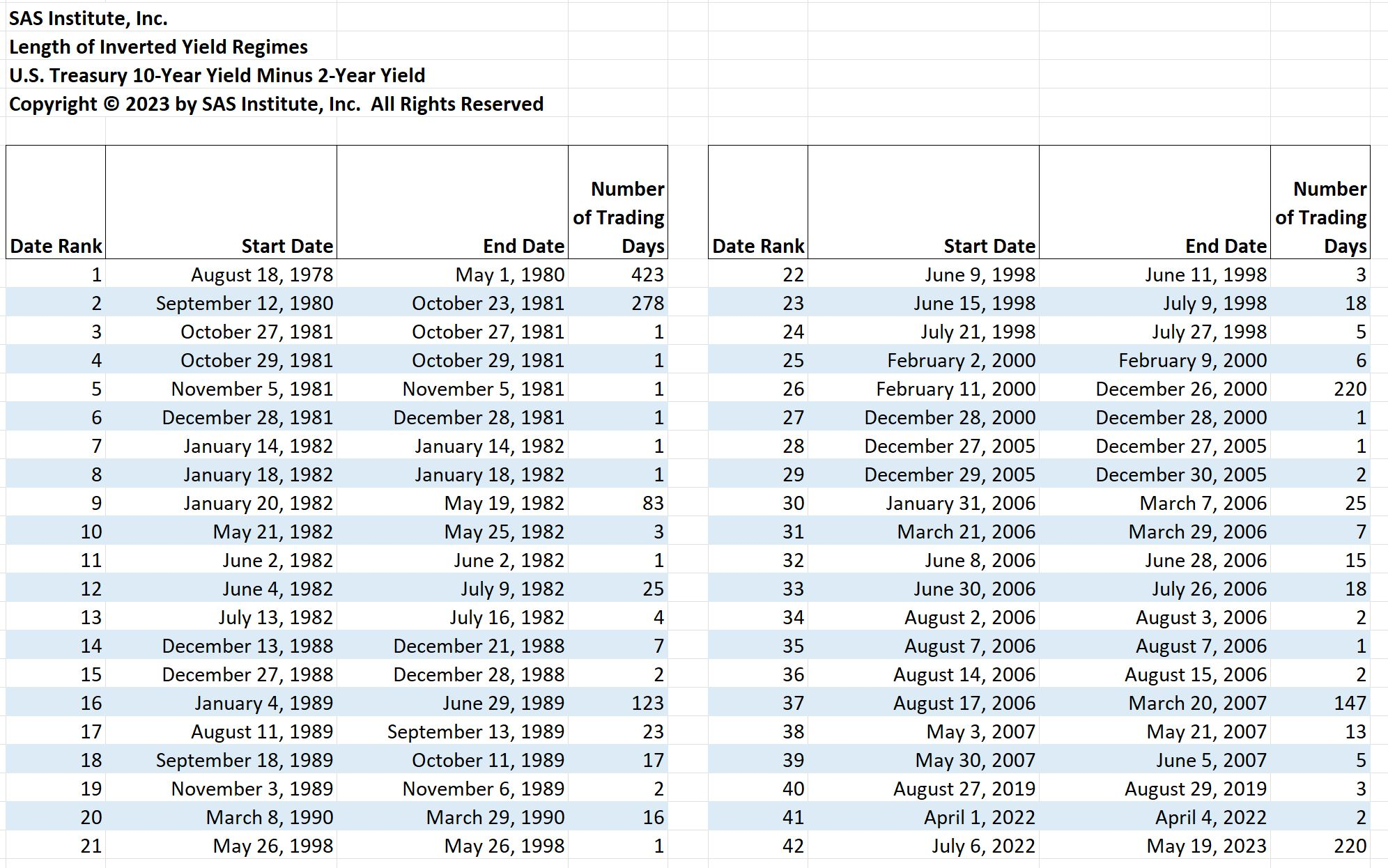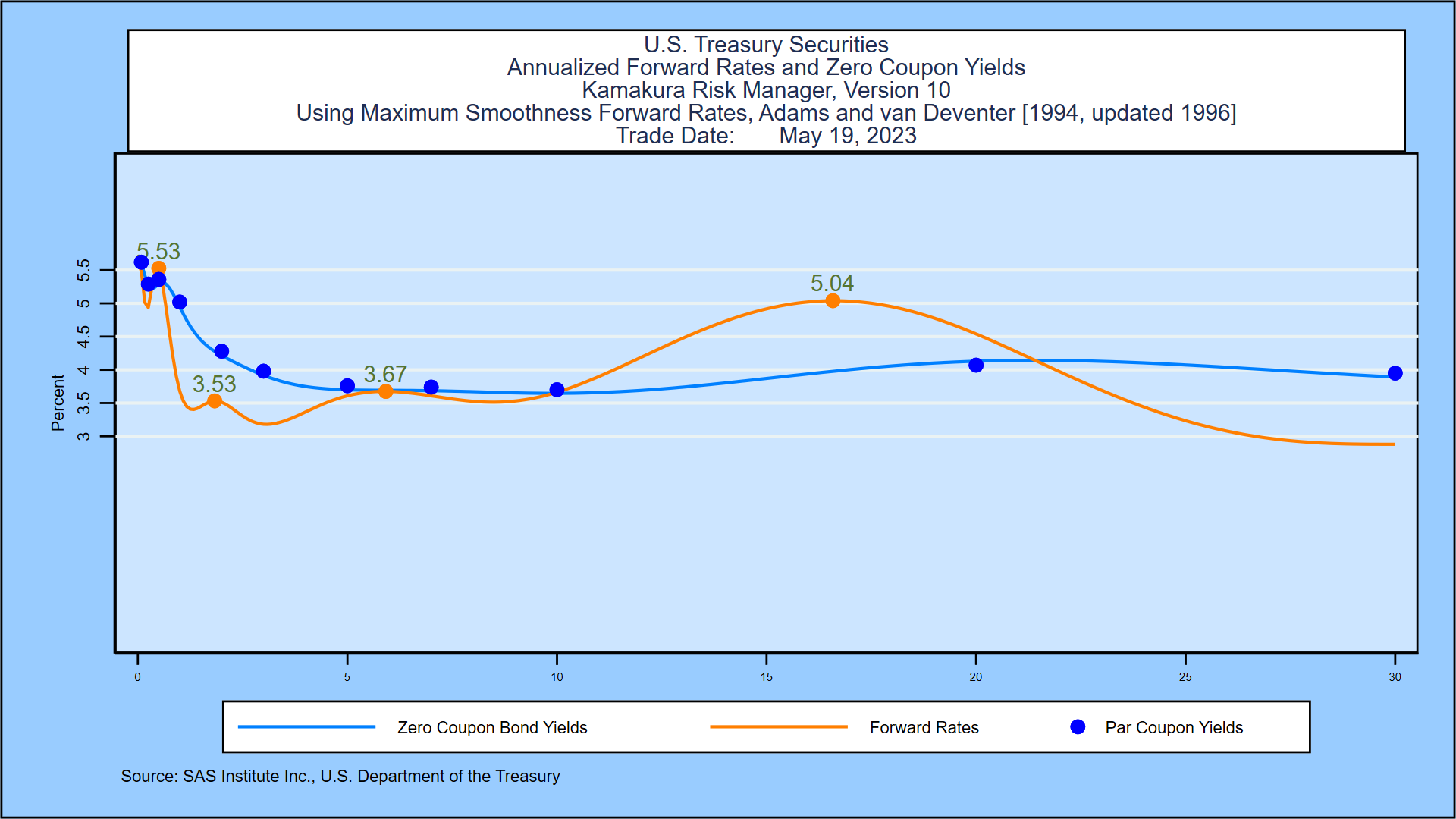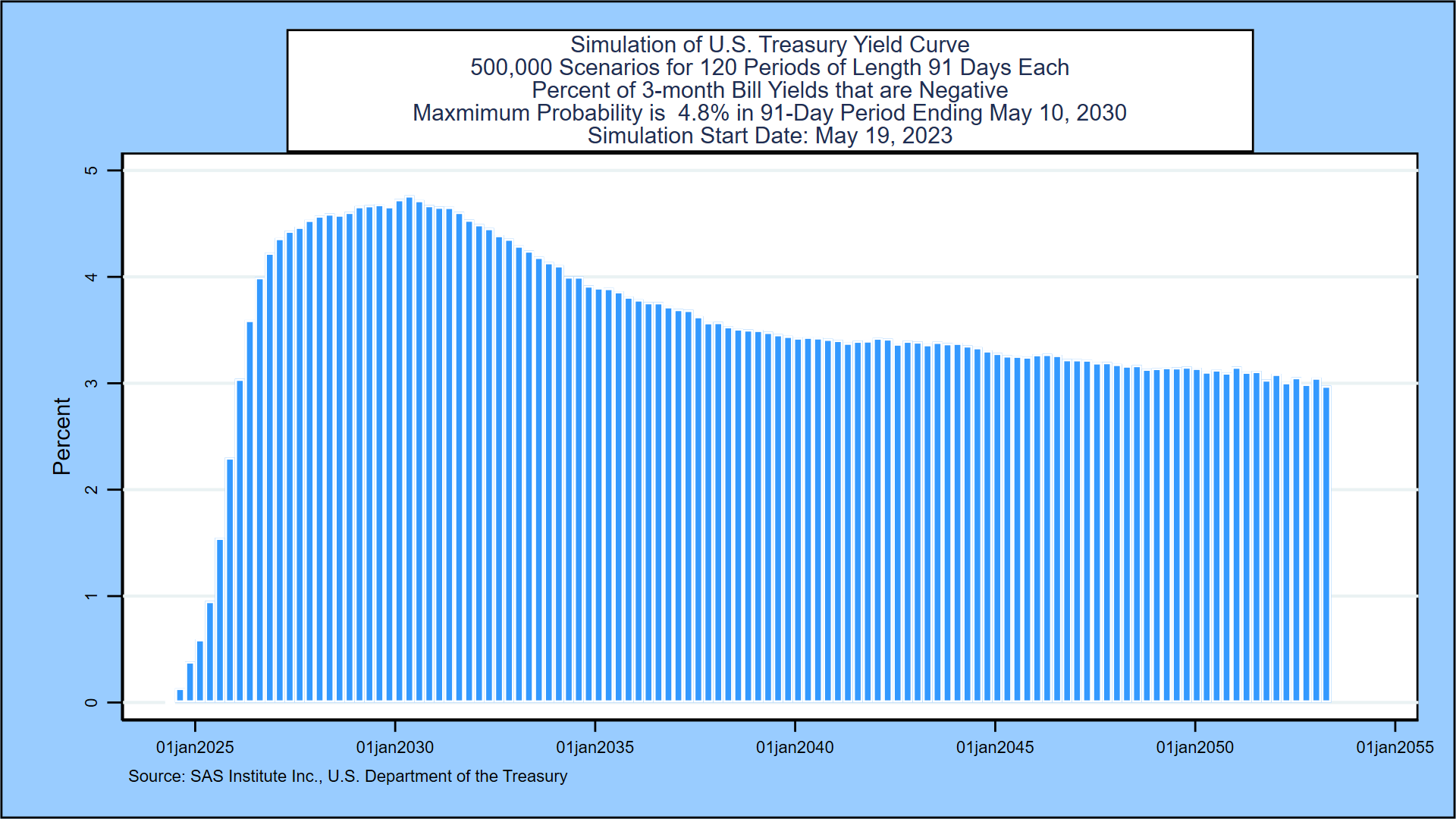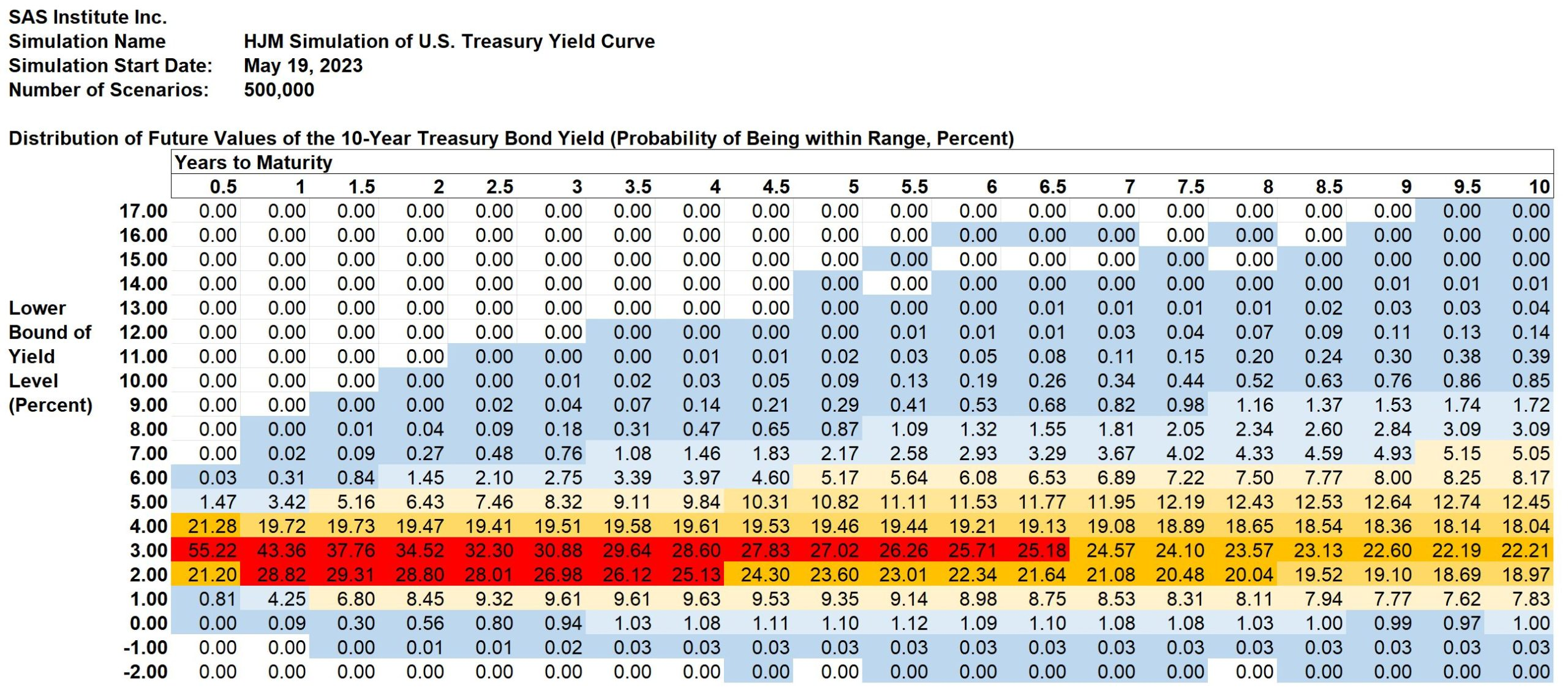Select PageDonald R. Van Deventer, Ph.D.

Don founded Kamakura Corporation in April 1990 and currently serves as its chairman and chief executive officer where he focuses on enterprise wide risk management and modern credit risk technology. His primary financial consulting and research interests involve the practical application of leading edge financial theory to solve critical financial risk management problems. Don was elected to the 50 member RISK Magazine Hall of Fame in 2002 for his work at Kamakura.

# SAS Weekly Forecast, May 19, 2023: Inverted Yield Streak Tied for Third Longest Since 1976

05/22/2023 04:26 AM

As of Friday, the current streak of trading days with a negative 2-year/10-year Treasury spread has reached 220 days, tied with the streak that ended in December, 2000 for third longest. Both implied forward Treasury 1-month bill rates and simulated 3-month bill rates show a sharp drop in the second semi-annual period in today’s simulation. The probability that the inverted yield curve ends by November 17, 2023 is now 19.5% compared to 27.6% last week. As explained in Prof. Robert Jarrow’s book cited below, forward rates contain a risk premium above and beyond the market’s expectations for the 1-month forward rate. We document the size of that risk premium in this graph, which shows the zero-coupon yield curve implied by current Treasury prices compared with the annualized compounded yield on 3-month Treasury bills that market participants would expect based on the daily movement of Treasury yields since 1962. The risk premium, the reward for a long-term investment, is large but narrowing over the full maturity range to 30 years. The graph also shows a sharp downward shift in yields in the first few years, as explained below.For more on this topic, see the analysis of U.S. Treasury yields through March 31, 2023 given in the appendix.

Inverted Yields, Negative Rates, and U.S. Treasury Probabilities 10 Years Forward

The negative 2-year/10-year Treasury spread has now persisted for 220 trading days, currently at a negative 58 basis points compared to negative 52 last week. The table below shows that the current streak of inverted yield curves is tied for the third longest in the U.S. Treasury market since the 2-year Treasury yield was first reported on June 1, 1976:In this week’s forecast, the focus is on three elements of interest rate behavior: the future probability of the recession-predicting inverted yield curve, the probability of negative rates, and the probability distribution of U.S. Treasury yields over the next decade.

We start from the closing U.S. Treasury yield curve and the interest rate swap quotations based on the Secured Overnight Financing Rate published daily by the Federal Reserve Bank of New York. Using a maximum smoothness forward rate approach, Friday’s implied forward rate curve shows a quick rise in 1-month rates to an initial peak of 5.53%, versus 5.79% last week. After the initial rise, there is substantial volatility and rates peak again at 3.67%, compared to 3.44% one week ago. Rates finally peak again at 5.04%, compared to 4.80% last week, and then decline to a lower plateau at the end of the 30-year horizon.Using the methodology outlined in the appendix, we simulate 500,000 future paths for the U.S. Treasury yield curve out to thirty years. The next three sections summarize our conclusions from that simulation.

Inverted Treasury Yields: Inverted Now, 80.5% Probability by November 17, 2023

A large number of economists have concluded that a downward sloping U.S. Treasury yield curve is an important indicator of future recessions. A recent example is this paper by Alex Domash and Lawrence H. Summers. We measure the probability that the 10-year par coupon Treasury yield is lower than the 2-year par coupon Treasury for every scenario in each of the first 80 quarterly periods in the simulation. The next graph shows that the probability of an inverted yield remains high, peaking at 80.5%, compared to 72.4% one week before, in the 91-day quarterly period ending November 17, 2023.Negative Treasury Bill Yields: 4.8% Probability by May 10, 2030

The next graph describes the probability of negative 3-month Treasury bill rates for all but the first 3 months of the next 3 decades. The probability of negative rates starts near zero but spikes, declines and then rises steadily to peak at 4.8%, compared to 5.1% one week earlier, in the period ending May 10, 2030:Calculating the Default Risk from Interest Rate Maturity Mismatches

In light of the interest-rate-risk-driven failure of Silicon Valley Bank on March 10, 2023, we have added a table that applies equally well to bank or institutional interest rate mismatches or individual mismatches from buying long-term Treasury bonds on margin. We assume that the sole asset is a 10-year Treasury bond purchased at time zero at par value of \$100. We analyze default risk for four different initial market value of equity to market value of asset ratios: 5%, 10%, 15%, and 20%. For the banking example, we assume that the only class of liabilities is deposits that can be withdrawn at par at any time. In the institutional and retail investor case, we assume that the liability is essentially a borrowing on margin/repurchase agreement with the possibility of margin calls. For all investors, the amount of liabilities (95, 90, 85 or 80) represents a “strike price” on a put option held by the liability holders. Failure occurs via a margin call, bank run, or regulatory take-over (in the banking case) when the value of assets falls below the value of liabilities.

The chart below shows the cumulative 10-year probabilities of failure for each of the 4 possible capital ratios when the asset’s maturity is 10 years. For the 5 percent case, that default probability is 40.94%, compared to 41.69% from last week.This default probability analysis is updated weekly based on the U.S. Treasury yield simulation described in the next section. The calculation process is the same for any portfolio of assets with credit risk included for many asset classes.

U.S. Treasury Probabilities 10 Years Forward

In this section, the focus turns to the decade ahead. This week’s simulation shows that the most likely range for the 3-month U.S. Treasury bill yield in ten years is from 1% to 2%. There is a 23.25% probability that the 3-month yield falls in this range, a change from 23.80% one week before. Note the significant shift downward in the second semi-annual period. For the 10-year Treasury yield, the most likely range is from 3% to 4%. The probability of being in this range is 22.21%, compared to 22.21% one week prior.

In a recent post on SeekingAlpha, we pointed out that a forecast of “heads” or “tails” in a coin flip leaves out critical information. What a sophisticated bettor needs to know is that, on average for a fair coin, the probability of heads is 50%. A forecast that the next coin flip will be “heads” is literally worth nothing to investors because the outcome is purely random.

The same is true for interest rates.

In this section we present the detailed probability distribution for both the 3-month Treasury bill rate and the 10-year U.S. Treasury yield 10 years forward using semi-annual time steps. We present the probability of where rates will be at each time step in 1 percent “rate buckets.” The forecast for 3-month Treasury yields is shown in this graph:3-Month U.S. Treasury Yield Data:

SAS3monthUST20230519

The probability that the 3-month Treasury bill yield will be between 1% and 2% in 2 years is shown in column 4: 27.69%. The probability that the 3-month Treasury bill yield will be negative (as it has been often in Europe and Japan) in 2 years is 1.50% plus 0.05% plus 0.00% = 1.55% (difference due to rounding). Cells shaded in blue represent positive probabilities of occurring, but the probability has been rounded to the nearest 0.01%. The shading scheme works like this:

• Dark blue: the probability is greater than 0% but less than 1%
• Light blue: the probability is greater than or equal to 1% and less than 5%
• Light yellow: the probability is greater than or equal to 5% and 10%
• Medium yellow: the probability is greater than or equal to 10% and less than 20%
• Orange: the probability is greater than or equal to 20% and less than 25%
• Red: the probability is greater than 25%

The chart below shows the same probabilities for the 10-year U.S. Treasury yield derived as part of the same simulation.10-Year US Treasury Yield Data:

SAS10yearUST20230519

Appendix: Treasury Simulation Methodology

The probabilities are derived using the same methodology that SAS Institute Inc. recommends to its KRIS® and Kamakura Risk Manager® clients, who currently have more than \$38 trillion in assets or assets under management. A moderately technical explanation is given later in the appendix, but we summarize it in plain English first.

Step 1: We take the closing U.S. Treasury yield curve as our starting point.

Step 2: We use the number of points on the yield curve that best explains historical yield curve shifts. Using daily data from 1962 through March 31, 2023, we conclude that 10 “factors” drive almost all movements of U.S. Treasury yields.

Step 3: We measure the volatility of changes in those factors and how it has changed over the same period.

Step 4: Using those measured volatilities, we generate 500,000 random shocks at each time step and derive the resulting yield curve.

Step 5: We “validate” the model to make sure that the simulation EXACTLY prices the starting Treasury curve and that it fits history as well as possible. The methodology for doing this is described below.

Step 6: We take all 500,000 simulated yield curves and calculate the probabilities that yields fall in each of the 1% “buckets” displayed in the graph.

Do Treasury Yields Accurately Reflect Expected Future Inflation?

We showed in a recent post on SeekingAlpha that, on average, investors have almost always done better by buying long term bonds than by rolling over short term Treasury bills. That means that market participants have generally (but not always) been accurate in forecasting future inflation and adding a risk premium to that forecast.

The distribution above helps investors estimate the probability of success from going long.

Finally, as mentioned weekly in The Corporate Bond Investor Friday overview, the future expenses (both the amount and the timing) that all investors are trying to cover with their investments are an important part of investment strategy. The author follows his own advice: cover the short-term cash needs first and then step out to cover more distant cash needs as savings and investment returns accumulate.

Technical Details

Daily Treasury yields form the base historical data for fitting the number of yield curve factors and their volatility. The historical data is provided by the U.S. Department of the Treasury.

An example of the modeling process using data through March 31, 2023 is available at this link.

The modeling process was published in a very important paper by David Heath, Robert Jarrow and Andrew Morton in 1992:Professor Jarrow’s biography is available here.

For technically inclined readers, we recommend Prof. Jarrow’s book Modeling Fixed Income Securities and Interest Rate Options for those who want to know exactly how the “HJM” model construction works.The number of factors (10 for the United States) has been stable for some time, but the volatilities for each factor are updated monthly and available to subscribers to the KRIS interest rate and macro-factor data service.

Footnotes:

 After the first 20 years in the simulation, the 10-year Treasury cannot be derived from the initial 30 years of Treasury yields.